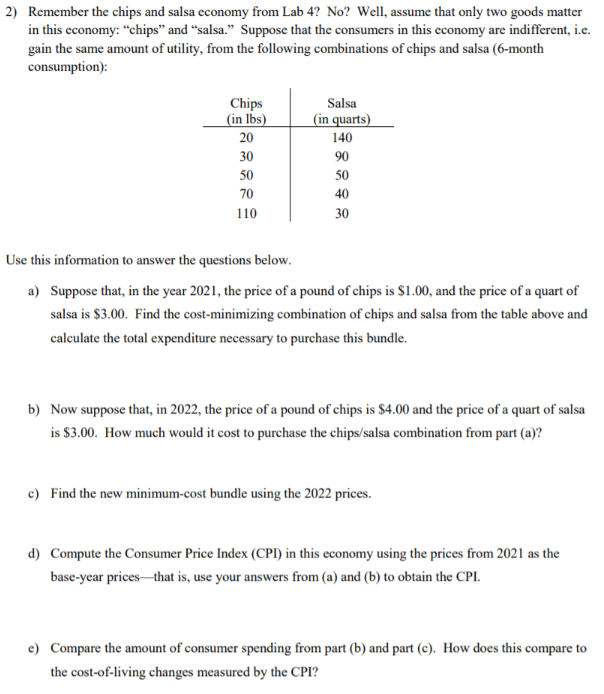# 2)Remember the chips and salsa economy from Lab 4? No? Well, assume that only two goods matterin this economy: "chips" and "salsa." Suppose that the consumers in this economy are indifferent, i.egain the same amount of utility, from the following combinations of chips and salsa (6-monthconsumption):Chips(in lbs)Salsa(in quarts)2014030905050704011030Use this information to answer the questions below.Suppose that, in the year 2021, the price of a pound of chips is \$1.00, and the price of a quart ofa)salsa is \$3.00. Find the cost-minimizing combination of chips and salsa from the table above andcalculate the total expenditure necessary to purchase this bundle.Now suppose that, in 2022, the price of a pound of chips is \$4.00 and the price of a quart of salsab)is \$3.00. How much would it cost to purchase the chips/salsa combination from part (a)?c)Find the new minimum-cost bundle using the 2022 prices.d)Compute the Consumer Price Index (CPI) in this economy using the prices from 2021 as thebase-year prices that is, use your answers from (a) and (b) to obtain the CPI.Compare the amount of consumer spending from part (b) and part (c). How does this compare toe)the cost-of-living changes measured by the CPI?

Question
16 views

d) and e)help_outlineImage Transcriptionclose2) Remember the chips and salsa economy from Lab 4? No? Well, assume that only two goods matter in this economy: "chips" and "salsa." Suppose that the consumers in this economy are indifferent, i.e gain the same amount of utility, from the following combinations of chips and salsa (6-month consumption): Chips (in lbs) Salsa (in quarts) 20 140 30 90 50 50 70 40 110 30 Use this information to answer the questions below. Suppose that, in the year 2021, the price of a pound of chips is \$1.00, and the price of a quart of a) salsa is \$3.00. Find the cost-minimizing combination of chips and salsa from the table above and calculate the total expenditure necessary to purchase this bundle. Now suppose that, in 2022, the price of a pound of chips is \$4.00 and the price of a quart of salsa b) is \$3.00. How much would it cost to purchase the chips/salsa combination from part (a)? c) Find the new minimum-cost bundle using the 2022 prices. d) Compute the Consumer Price Index (CPI) in this economy using the prices from 2021 as the base-year prices that is, use your answers from (a) and (b) to obtain the CPI. Compare the amount of consumer spending from part (b) and part (c). How does this compare to e) the cost-of-living changes measured by the CPI? fullscreen
check_circle

Step 1

(d) Consumer price index (CPI) measures the change in the price for a particular basket of good and can be calculated as the ratio of cost of basket in the given year and cost of basket in the base year.

From p...

### Want to see the full answer?

See Solution

#### Want to see this answer and more?

Solutions are written by subject experts who are available 24/7. Questions are typically answered within 1 hour.*

See Solution
*Response times may vary by subject and question.
Tagged in